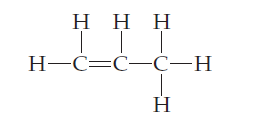×
Get Full Access to Chemistry: The Central Science - 12 Edition - Chapter 9 - Problem 63e
Get Full Access to Chemistry: The Central Science - 12 Edition - Chapter 9 - Problem 63e

×

# Multiple Bonds (Section)Propylene, C3H6, is a gas that isISBN: 9780321696724 27

## Solution for problem 63E Chapter 9

Chemistry: The Central Science | 12th Edition

• Textbook Solutions
• 2901 Step-by-step solutions solved by professors and subject experts
• Get 24/7 help from StudySoup virtual teaching assistantsChemistry: The Central Science | 12th Edition

4 5 1 289 Reviews
30
2
Problem 63E

Propylene, C3H6, is a gas that is used to form the important polymer called polypropylene. Its Lewis structure is(a)What is the total number of valence electrons in the propylene molecule? (b) How many valence electrons are used to make σ bonds in the molecule? (c) How many valence electrons are used to make 𝜋 bonds in the molecule? (d) How many valence electrons remain in nonbonding pairs in the molecule? (e) What is the hybridization at each carbon atom in the molecule?

Step-by-Step Solution:
Step 1 of 3

Cell Biology BIOL 225 Chapter 2 – The Chemical Basis of Life Important Functional Groups to Consider Types of Bonds • Covalent Bonds – Sharing of electrons, strong bonds, 80-­‐100 kcal/mol to br

Step 2 of 3

Step 3 of 3

##### ISBN: 9780321696724

The full step-by-step solution to problem: 63E from chapter: 9 was answered by , our top Chemistry solution expert on 04/03/17, 07:58AM. This full solution covers the following key subjects: molecule, electrons, valence, bonds, used. This expansive textbook survival guide covers 49 chapters, and 5471 solutions. Since the solution to 63E from 9 chapter was answered, more than 438 students have viewed the full step-by-step answer. Chemistry: The Central Science was written by and is associated to the ISBN: 9780321696724. The answer to “?Propylene, C3H6, is a gas that is used to form the important polymer called polypropylene. Its Lewis structure is (a)What is the total number of valence electrons in the propylene molecule? (b) How many valence electrons are used to make ? bonds in the molecule? (c) How many valence electrons are used to make ???? bonds in the molecule? (d) How many valence electrons remain in nonbonding pairs in the molecule? (e) What is the hybridization at each carbon atom in the molecule?” is broken down into a number of easy to follow steps, and 83 words. This textbook survival guide was created for the textbook: Chemistry: The Central Science, edition: 12.

Unlock Textbook Solution